### Iteration Formulas

The iteration formula is the main formula for fractals: This formula is responsible for the complexity of the fractal, i.e. the detail level and its shape.
Have a look at the following three images: They all show the same area using exactly the same settings except the iteration formula.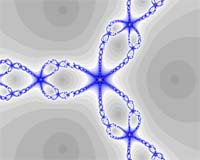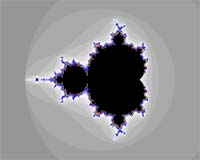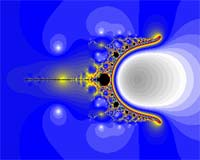Ok, now let’s see how you actually assign an iteration formula to a fractal. All settings are concentrated in the parameter window, tab “formula” (see below).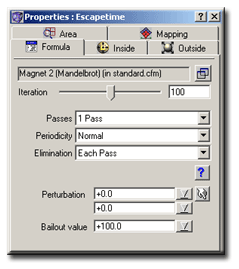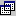Setting a Formula: By pressing this button a modal dialog box will appear: It will display all formulas currently known to ChaosPro. Select a formula and double click onto the formula name to assign it to the formula. If you hold down the “SHIFT”-key on your keyboard, the current parameter values are preserved and the new formula will use that values. Iteration: This value specifies the maximum number of iterations which ChaosPro should perform. After each iteration the so called “bail-out” condition is evaluated: If it evaluates to true, the loop exits and the pixel is considered to belong to the “outer” area. If the loop was executed the maximum number of iterations, then it terminates, too, but the pixel is considered to belong to the “inner” area. The beauty of the fractals mainly comes from the border between the “inner” area and the “outer” area: This border can be excitingly complicated. Passes: This item lets you specify the number of passes used to draw the image. So if you choose 3 Passes, then at first only every 4th pixel (horizontally and vertically) will be calculated and the image will consist of 4×4 blocks (and thus will look quite clumsy). But the advantage is, that a first preview is finished in 1/16 of the total time, so you quickly have an imagination of how the image will look like at the end. After this first pass the second pass calculates every 2nd pixel, and the third pass will calculate all remaining pixels.
• Periodicity checking speeds up the calculation by examining the iteration loop and searching for fixed points and similar attractors. In that case ChaosPro knows that the maximum number of iterations will be reached at the end, and thus it can stop the process.
This speeds up the calculation, but as you can imagine, the program can guess wrong: In that case, the final image contains sections which seem to be “inside”. Have a look at the following three example images: Below each image you can find the time needed and the setting of “Perodicity Check”.Check=Off Calculation time: 12.3 secCheck=Normal Calculation time: 8.3 secCheck=Very Soft Calculation time: 7.3 sec

As you can see, the proper setting of “Periodicity Check” speeds up the calculation enourmously, but you will need to manually verify whether the setting is suitable. Some fractal formulas work perfectly with periodicity checking logic, whereas others won’t work at all…

• Elimination: This setting specifies whether ChaosPro should guess pixel colors between subsequent draw passes: This can speed up calculation times at the cost of accuracy. I suggest to enable it for exploring, because it makes fractals calculate faster. But at the end you should set it to “off” in order to see whether there is any difference. If not, switch it on again…

If you have chosen an iteration formula you can see that the lower part of the window will display additional parameters: It contains the parameters of the formula as defined by the author of that formula. To read more about formula parameters and the user interface provided to them read User Interface.